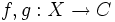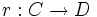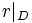# Convex subset of Euclidean space

## Definition

A convex subset of Euclidean space is a subset in$\R^n$ for some$n$, with the property that given any two points in the subset, the line segment joining those two points also lies completely within the subset.

Note that in place of a finite-dimensional Euclidean space$\R^n$, we can also apply this definition to an infinite-dimensional Euclidean space, where it is also very useful.

## Facts

### Any two functions to a convex subset are linearly homotopic

If$C$ is a convex subset of$\R^n$ and$f,g:X \to C$ are continuous functions, then we can define a linear homotopy from$f$ to$g$, and hence$f$ and$g$ are homotopic.

### Any retract is a deformation retract

Suppose$C$ is a convex subset and$r:C \to D$ is a retraction (viz,$r$ is a continuous map from$C$ to$D$ such that$r|_D$ is the identity map. Then the linear homotopy between the identity map on$C$ and the function$r$ is a deformation retraction from$C$ to$D$.

### The space is contractible in a semi-sudden way

A convex subset can be contracted to any point in it, by the linear homotopy. The linear homotopy has the further interesting property that for$t < 1$, it gives a homeomorphism to its image (since it's just dilation by a factor). Thus, it is a semi-sudden homotopy.